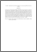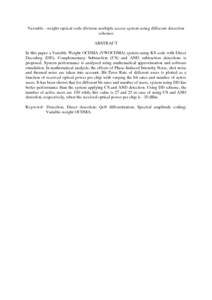# Variable - weight optical code division multiple access system using different detection schemes

## Citation

Seyedzadeh, Saleh and Moghaddasi, Majid and A. Anas, Siti B. (2016) Variable - weight optical code division multiple access system using different detection schemes. Journal of Telecommunications and Information Technology, 3 (50). pp. 50-59. ISSN 1509-4553

## Abstract

In this paper a Variable Weight OCDMA (VWOCDMA) system using KS code with Direct Decoding (DD), Complementary Subtraction (CS) and AND subtraction detections is proposed. System performance is analyzed using mathematical approximation and software simulation. In mathematical analysis, the effects of Phase-Induced Intensity Noise, shot noise and thermal noise are taken into account. Bit Error Rate of different users is plotted as a function of received optical power per chip with varying the bit rates and number of active users. It has been shown that for different bit rates and number of users, system using DD has better performance than the system applying CS and AND detection. Using DD scheme, the number of active users are 100 while this value is 27 and 25 in case of using CS and AND detection, respectively, when the received optical power per chip is –10 dBm.Preview
PDF (Abstract)
Variable - weight optical code division multiple access system using different detection schemes.pdfView Item